Paul Erdös和Noga Alon等人给出了一般意义的Ramsey数理论，本文通过概率方法给出了高维情况下的Ramsey数理论及其推广的一般形式。给出了等概率2-着色情况：当k=lRd(k,k)的下界结果；当kl有区分时Rd(l,k)的下界结果。给出了等概率3-着色情况：当k=l=mRd(k,k,k)的下界结果；当k,l,m有区分时Rd(l,k,m)的下界结果。给出了r-着色情况下：当k1=k2=...=krRd(k,k,...k)的下界结果；当k1,k2,...,kr有区分时Rd(k1,k2,...,kr)的下界结果。最后又给出了不等概率时上述各种情况下的相应结果。 Paul Erdös and Noga Alon give a general sense of Ramsey Number Theory. This paper presents a general form of Ramsey Number Theory in the dimensional case and its promotion by using probabilistic methods. The paper shows the lower bound of Rd(k,k) in the case of k=l with equal probabilistic 2-coloring situation, and shows the lower bound of Rd(l,k) in the case of k and l distinguishing with equal probabilistic 2-coloring situation. The paper shows the lower bound of Rd(k,k,k) in the case of k=l=m with equal probabilistic 3-coloring situation, and shows the lower bound of Rd(l,k,m) in the case of k, l and m distinguishing with equal probabilistic 3-coloring situation. And the paper shows the lower bound of Rd(k,k,...k) in the case of k1=k2=...=kr with equal probabilistic r-coloring situation, and shows the lower bound of Rd(k1,k2,...,kr) in the case of k1, k2,...,kr distinguishing with equal probabilistic r-coloring situation. Finally, we also give the corresponding results of the variety of situations with unequal probabilities.

Email: S201306040@emails.bjut.edu.cn

Paul Erdös和Noga Alon等人给出了一般意义的Ramsey数理论，本文通过概率方法给出了高维情况下的Ramsey数理论及其推广的一般形式。给出了等概率2-着色情况：当的下界结果；当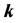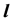有区分时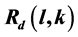的下界结果。给出了等概率3-着色情况：当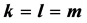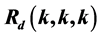的下界结果；当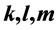有区分时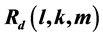的下界结果。给出了r-着色情况下：当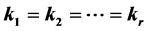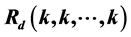的下界结果；当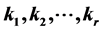有区分时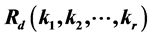的下界结果。最后又给出了不等概率时上述各种情况下的相应结果。

1. 引言